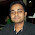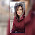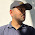# Frequency Modulation (FM) Simulation / Generation Using MATLAB Code

Frequency Modulation using MATLAB
Frequency modulation (FM) is the encoding of information in a carrier wave by varying the instantaneous frequency of the wave. The amount of frequency variation is proportional to the Modulation index.
A major advantage of FM as compared  with AM, is improved Signal-to-noise ratio (SNR). The improvement depends on modulation level and deviation. For typical voice communications channels, improvements are typically 5-15 dB.

The following MATLAB code for generating/simulating the Frequency Modulation requires 5 inputs, amplitude of both message & signal, frequency of both message & signal & the modulation index.

MATLAB CODE For FM (Frequency Modulation)

clc
clear all
close all
t = 0:0.001:1;
%upto 1000 samples
vm = input('Enter Amplitude (Message) = ');
vc = input('Enter Amplitude (Carrier) = ');
fM = input('Enter Message frequency = ');
fc = input('Enter Carrier frequency = ');
m = input('Enter Modulation Index = ');
msg = vm*sin(2*pi*fM*t);
subplot(3,1,1);
%plotting message signal
plot(t,msg);
xlabel('Time');
ylabel('Amplitude');
title('Message ');

carrier = vc*sin(2*pi*fc*t);
subplot(3,1,2);
%plotting carrier signal
plot(t,carrier);
xlabel('Time');
ylabel('Amplitude');
title('Carrier Signal');

y = vc*sin(2*pi*fc*t+m.*cos(2*pi*fM*t));
subplot(3,1,3);
%plotting FM (Frequency Modulated) signal
plot(t,y);
xlabel('Time');
ylabel('Amplitude');
title('FM Signal');

Sample MATLAB Input:
Enter Amplitude (Message) = 5
Enter Amplitude (Carrier) = 5
Enter Message frequency = 8
Enter Carrier frequency = 100
Enter Modulation Index = 10

MATLAB's Output For The Above Input:Frequency Modilation (FM) Simulation / Generation Using MATLAB Code
#Matlab Christmas Tree Plot- Christmas celebration through matlab way - Merry Christmas!

1.what is the reason for phase shift in output

1.You tried a demodulation?

2.Did u solve that problem? ?

2.There supposed to be 3 frequencies - lowest , 0 , highest . Two are visible here!

1.Hi. If you look at the spectrum of fm signal you will find multiple peaks spread across the carrier frequency. So there are not only 3 frequencies you will see, but multiple. I have used this formula of, y = vc*sin(2*pi*fc*t+m.*cos(2*pi*fM*t)); to get a FM signal. If you look for FM's theoretical expressions for comparison. You will find that, delta_f=m*fm/Am. Once you found that, then by using Carson's rule you can obtain the bandwidth. After calculating the bandwidth you can decide the lowest and highest frequency.